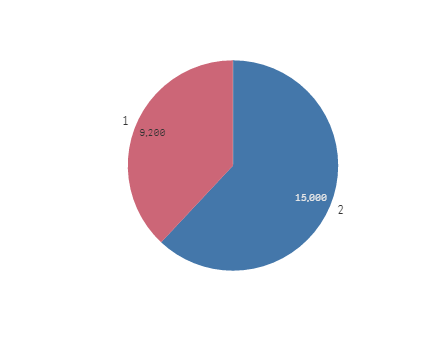# Qlik Sense App Development

Announcements
See why BI users voted Qlik #1 in 11 categories. GET REPORT
cancel
Showing results for
Did you mean:
HighlightedCreator III

## Create a Calculated Pareto Dimension for a Pie Chart

Hello all! I have a dataset that is as follows:

Vendor       Sales    Type

A              1300        C

B              2200        N

A              1400        N

B              2100        N

C              2500        C

A              300          N

C              900          C

D              3200        N

B              2000        C

D              1500        C

E              1100        N

F              3500        C

E              1500        C

G              1400        N

H              600          C

H              900          N

G             1200         C

Now I would like to create a Calculated dimension to use in a Pie chart. The Pie chart should have the Dimensions as 'C' and 'N Top 80%'. To further break it down, I'd like to show the % of Sales coming under the Type C and % of Sales coming under the Top 80%(Pareto) Vendors in Type N. Can you please help me create a calculated dimension to achieve this? TIA!

1 Solution

Accepted Solutions
HighlightedMVP

Something like this?Dimension

=ValueList(1, 2)

Expression

Pick(ValueList(1, 2),

Sum(Aggr(If(Rangesum(Above(Sum({<Vendor, Type = {'N'}>} Sales)/Sum({<Vendor, Type = {'N'}>} TOTAL Sales),0,RowNo()))<=0.8, Sum({<Type = {'N'}>}Sales)), (Vendor,(=Sum({<Vendor, Type = {'N'}>} Sales),Desc)))),

Sum({<Type = {'C'}>}Sales))

16 Replies
HighlightedMVP

Can you put down the result you expect to see from this data in a tabular form for us to understand your requirement?

HighlightedCreator III

Sure. Now what I'd like for the Pie chart to have is two partitions: Sales under Type 'C' and Sales coming from Top 80% Vendors in Type N. If we perform a Pareto analysis on the above dataset within Type N, we can see that the Vendors 'A','B' and 'D' fall into the Top 80% category. So the result should display as follows: 9200 Under Top 80% Type N

Vendor Split: A:1700(1400+300),

B:4300(2100+2200),

D:3200) and

15000 under Type C:

(Vendor Split:A:1300,

B:2000,

C:3400(2500+900),

D:1500,

E:1500,

F:3500,

G:1200,

H:600).

HighlightedMVP

Something like this?Dimension

=ValueList(1, 2)

Expression

Pick(ValueList(1, 2),

Sum(Aggr(If(Rangesum(Above(Sum({<Vendor, Type = {'N'}>} Sales)/Sum({<Vendor, Type = {'N'}>} TOTAL Sales),0,RowNo()))<=0.8, Sum({<Type = {'N'}>}Sales)), (Vendor,(=Sum({<Vendor, Type = {'N'}>} Sales),Desc)))),

Sum({<Type = {'C'}>}Sales))

HighlightedCreator III

As usual you are correct! Could you please explain a little bit about the expression and the ValueList function or give the relevant links? Much much appreciated! Thanks a lot!

HighlightedMVP

Valuelist is a synthetic dimension. I can go over its explanation, but some of the other guys have already done the great work. Check these links out

ValueList() – For those tricky situations

Missing Manual - ValueLoop() &amp; ValueList()

ValueList - chart function ‒ Qlik Sense

HighlightedCreator III

Sorry for the trouble but let's say there's another field for the data as follows:

Vendor       Sales    Type    Type1

A              1300        C           M

B              2200        N           S

A              1400        N           M

B              2100        N           M

C              2500        C           S

A              300          N           M

C              900          C           M

D              3200        N           M

B              2000        C           S

D              1500        C           S

E              1100        N           S

F              3500        C           M

E              1500        C          M

G              1400        N          S

H              600          C         M

H              900          N         S

G             1200         C         M

Now I'd like to display the same pie chart above for the Sales of in Bottom 20% Pareto of Type1='M'. How should I modify the above expression?

HighlightedMVP

How can you have two fields with Type1 header?

HighlightedCreator III